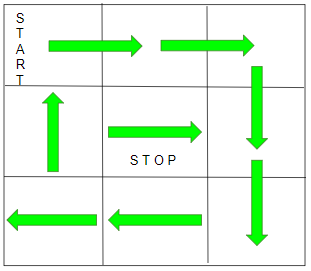# Final direction after visiting every cell of Matrix starting from (0, 0)

Given a 2D grid of size N x M. The task is to find the final direction after visiting every cell under given conditions.

• You can start only from the top left corner of the N*M grid and facing towards the right.
• You can walk one square at a time in the direction you are facing.
• If you reach the boundary of the grid or if the next square you are about to visit has already been visited, you turn right.

Examples:

Input: N = 3, M = 3
Output: Right
Explanation: Below is the final position after traversing the gridInput: N = 3, M = 1
Output: Down

## Recommended: Please try your approach on {IDE} first, before moving on to the solution.

Approach: For the above problem statement we have to observe the following:

• The path formed will always be a Spiral path. So, we can say that any of the middle cells will be the final cell and we need to find the direction of that cell.
• If n > m, then the final direction could only be Up or Down depending on the value of m because there always be some cell left in the last uncovered column when all other columns are covered.
• If n <= m, then the final direction could only be Left or Right depending on the value of n.

Therefore on the basis of above observations, there can be only 4 cases for 4 directions:

1. If n > m and m is even, final direction will be Up.
2. If n > m and m is odd, final direction will be Down.
3. If n <= m and n is even, final direction will be Left.
4. If n <= m and n is odd, final direction will be Right.

Below is the implementation of the above approach:

## C++

 `// C++ program to find the direction ` `// when stopped moving ` ` `  `#include ` `using` `namespace` `std; ` ` `  `// Function to find the direction ` `// when stopped moving ` `void` `findDirection(``int` `n, ``int` `m) ` `{ ` `    ``if` `(n > m) { ` `        ``if` `(m % 2 == 0) ` `            ``printf``(``"Up\n"``); ` `        ``else` `            ``printf``(``"Down\n"``); ` `    ``} ` `    ``else` `{ ` `        ``if` `(n % 2 == 0) ` `            ``printf``(``"Left\n"``); ` `        ``else` `            ``printf``(``"Right\n"``); ` `    ``} ` `} ` ` `  `// Driver Code ` `int` `main() ` `{ ` `    ``// Given size of NxM grid ` `    ``int` `n = 3, m = 3; ` ` `  `    ``// Function Call ` `    ``findDirection(n, m); ` `    ``return` `0; ` `} `

## Java

 `// Java program to find the direction  ` `// when stopped moving  ` `class` `GFG{  ` ` `  `// Function to find the direction  ` `// when stopped moving  ` `static` `void` `findDirection(``int` `n, ``int` `m)  ` `{  ` `    ``if` `(n > m)  ` `    ``{  ` `        ``if` `(m % ``2` `== ``0``)  ` `            ``System.out.print(``"Up\n"``);  ` `        ``else` `            ``System.out.print(``"Down\n"``);  ` `    ``}  ` `    ``else`  `    ``{  ` `        ``if` `(n % ``2` `== ``0``)  ` `            ``System.out.print(``"Left\n"``);  ` `        ``else` `            ``System.out.print(``"Right\n"``);  ` `    ``}  ` `}  ` ` `  `// Driver code  ` `public` `static` `void` `main(String[] args)  ` `{ ` `     `  `    ``// Given size of NxM grid  ` `    ``int` `n = ``3``, m = ``3``;  ` ` `  `    ``// Function Call  ` `    ``findDirection(n, m);  ` `}  ` `}  ` ` `  `// This code is contributed by shubham  `

## Python3

 `# Python3 program to find the direction ` `# when stopped moving ` ` `  `# Function to find the direction ` `# when stopped moving ` `def` `findDirection(n, m): ` ` `  `    ``if` `(n > m): ` `        ``if` `(m ``%` `2` `=``=` `0``): ` `            ``print``(``"Up\n"``); ` `        ``else``: ` `            ``print``(``"Down\n"``); ` `     `  `    ``else``: ` `        ``if` `(n ``%` `2` `=``=` `0``): ` `            ``print``(``"Left\n"``); ` `        ``else``: ` `            ``print``(``"Right\n"``); ` `     `  `# Driver Code ` ` `  `# Given size of NxM grid ` `n ``=` `3``; m ``=` `3``; ` ` `  `# Function Call ` `findDirection(n, m); ` ` `  `# This code is contributed by Code_Mech `

## C#

 `// C# program to find the direction  ` `// when stopped moving  ` `using` `System; ` `class` `GFG{  ` ` `  `// Function to find the direction  ` `// when stopped moving  ` `static` `void` `findDirection(``int` `n, ``int` `m)  ` `{  ` `    ``if` `(n > m)  ` `    ``{  ` `        ``if` `(m % 2 == 0)  ` `            ``Console.Write(``"Up\n"``);  ` `        ``else` `            ``Console.Write(``"Down\n"``);  ` `    ``}  ` `    ``else` `    ``{  ` `        ``if` `(n % 2 == 0)  ` `            ``Console.Write(``"Left\n"``);  ` `        ``else` `            ``Console.Write(``"Right\n"``);  ` `    ``}  ` `}  ` ` `  `// Driver code  ` `public` `static` `void` `Main()  ` `{ ` `     `  `    ``// Given size of NxM grid  ` `    ``int` `n = 3, m = 3;  ` ` `  `    ``// Function Call  ` `    ``findDirection(n, m);  ` `}  ` `}  ` ` `  `// This code is contributed by Code_Mech `

Output:

```Right
```

Time Complexity: O(1)
Auxiliary Space: O(1)

Attention reader! Don’t stop learning now. Get hold of all the important DSA concepts with the DSA Self Paced Course at a student-friendly price and become industry ready.

My Personal Notes arrow_drop_upCheck out this Author's contributed articles.

If you like GeeksforGeeks and would like to contribute, you can also write an article using contribute.geeksforgeeks.org or mail your article to contribute@geeksforgeeks.org. See your article appearing on the GeeksforGeeks main page and help other Geeks.

Please Improve this article if you find anything incorrect by clicking on the "Improve Article" button below.